Courses

NCERT Solutions - Cubes and Cubes Roots Notes - Class 8

Class 8: NCERT Solutions - Cubes and Cubes Roots Notes - Class 8

The document NCERT Solutions - Cubes and Cubes Roots Notes - Class 8 is a part of Class 8 category.
All you need of Class 8 at this link: Class 8

Exercise 7.1

Question 1: Which of the following numbers are not perfect cubes:

(i) 216

(ii) 128

(iii) 1000

(iv) 100

(v) 46656

(i) 216

Prime factors of 216 = 2x2x2x3x3x3

Here all factors are in groups of 3’s [in triplets) Therefore, 216 is a perfect cube number.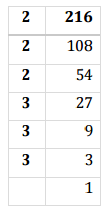(ii)

Prime factors of 128 =2x2x2x2x2x2x2

Here one factor 2 does not appear in a 3's group.

Therefore, 128 is not a perfect cube.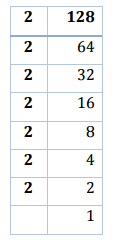(iii)

Prime factors of 1000 =2x2x2x3x3x3

Here all factors appear in 3's group.

Therefore, 1000 is a perfect cube.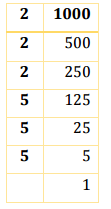(iv)

Prime factors of 100 =2x2x5x5

Here all factors do not appear in 3’s group.

Therefore, 100 is not a perfect cube.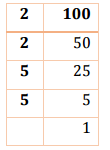(v)

Prime factors of 46656 = 2x2x2 x2x2x2x3x3x3x3x3x3

Here all factors appear in 3's group.

Therefore, 46656 is a perfect cube.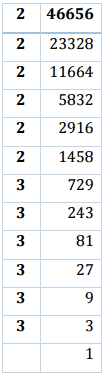Question 2:

Find the smallest number by which each of the following numbers must be multiplied to obtain a perfect cube:

(i) 243

(ii) 256

(iii) 72

(iv) 675

(v) 100

(i)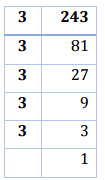Prime factors of 243 =3x3x3x3x3

Here 3 does not appear in 3's group.

Therefore, 243 must be multiplied by 3 to make it a perfect cube.

(ii)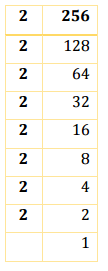Prime factors of 256 = 2 x 2 x 2 x 2x2x 2x2x2

Here one factor 2 is required to make a 3's group.

Therefore, 256 must be multipl ied by 2 to make it a perfect cube.

(iii)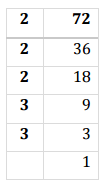Prime factors of 72 = 2x2x2x3x3

Here 3 does not appear in 3's group.

Therefore, 72 must be multiplied by 3 to make it a perfect cube.

(iv)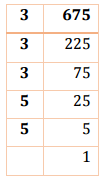Prime factors of 675 =3x3x3x5x5

Here factor 5 does not appear in 3's group.

Therefore 675 must be multiplied by 3 to make it a perfect cube.

(v)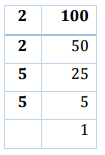Prime factors of l00 = 2x2x5x5

Here factor 2 and 5 both do not appear in 3's group.

Therefore 100 must be multiplied by 2 x 5 = 10 to make it a perfect cube

Question 3:

Find the smallest number by which each of the following numbers must be divided to obtain a perfect cube:

(i) 81

(ii) 128

(iii) 135

(iv) 192

(v) 704

(i)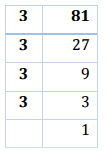Prime factors of 81 = 3x3x3x3

Here one factor 3 is not grouped in triplets.

Therefore 81 must be divided by 3 to make it a perfect cube.

(ii)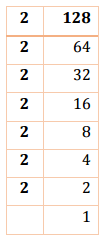Prime factors of 128 =2x2x2x2x2x2x2

Here one factor 2 does not appear in a 3's group.

Therefore, 128 must be divided by 2 to make it a perfect cube

(iii)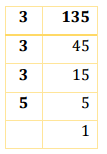Prime factors of 135 = 3x3x3x5

Here one factor 5 does not appear in a triplet.

Therefore, 135 must be divided by 5 to make it a perfect cube.

(iv)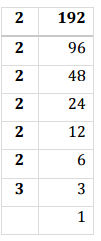Prime factors of 192 =2x2x2x2x2x2x3

Here one factor 3 does not appear in a triplet

Therefore, 192 must be divided by 3 to make it a perfect cube.

(v)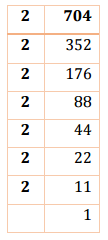Prime factors of 704 = 2x2x2x2x2x2x11

Here one factor 11 does not appear in a triplet

Therefore, 704 must be divided by 11 to make it a perfect cube.

Question 4:

Parikshit makes a cuboid of plasticine of sides 5 cm, 2 cm, 5 cm. How many such cuboids will he need to form a cube?

Given numbers = 5 x 2 x 5

Since, Factors of 5 and 2 both are not in group of three.

Therefore, the number must be multiplied by 2 x 2 x 5 = 20 to make it a perfect cube.

Hence he needs 20 cuboids.

Exercise 7.2

Question 1: Find the cube root of each of the following numbers by prime factorization method:

(i) 64

(ii) 512

(iii) 10648

(iv] 27000

(v) 15625

[vi] 13824

(vii) 110592

[viii] 46656

(ix) 175616

(x) 91125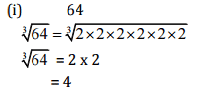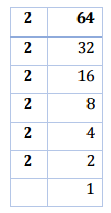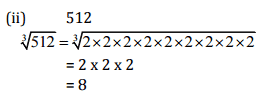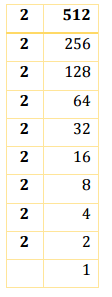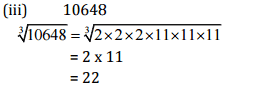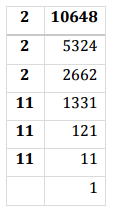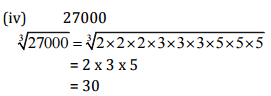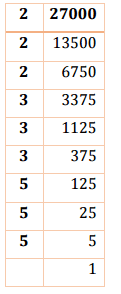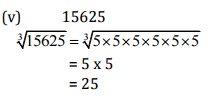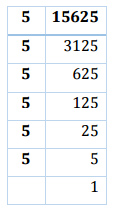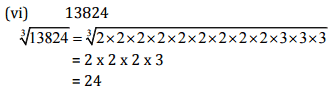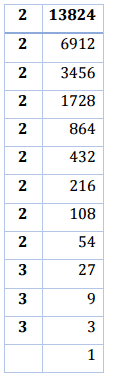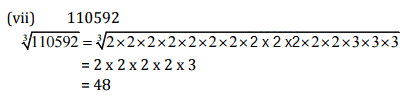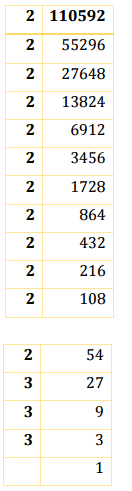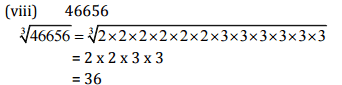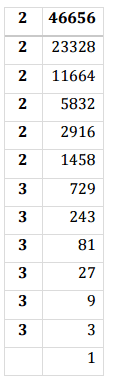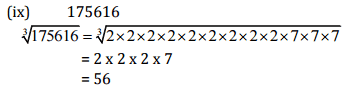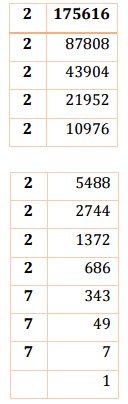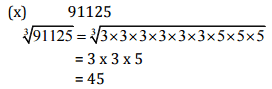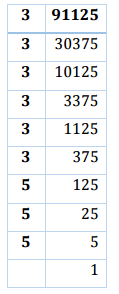Question 2:

State true or false:

(i) Cube of any odd number is even.
(ii) A perfect cube does not end with two zeroes.
(iii) If square of a number ends with 5, then its cube ends with 25.
(iv) There is no perfect cube which ends with 8.
(v) The cube of a two digit number may be a three digit number.
(vi) The cube of a two digit number may have seven or more digits.
(vii) The cube of a single digit number may be a single digit number.

(i) False

Since, 1'=1,3= 27,53 = 125,. ......... are all odd.

(ii) True

Since, a perfect cube ends with three zeroes.

e.g. 103 = 1000,203 =8000,303 = 27000,...... soon

(iii) False

Since, 52 = 25,53 = 125, 152 = 225,153 = 3375 [Did not end with 2 5)

(iv) False

Since 12=1728 [Ends with 8]

And 22=10648 [Ends with 8]

(v) False

Since 10=1000 [Four digit number]

And 113 =1331 [Four digit number]

[vi] False

Since 993 = 970299 [Six digit number]

(vii) True

13 =1 [Single digit number]

23 =8 [Single digit number]

Question 3:

You are told that 1,331 is a perfect cube. Can you guess with factorization what is its cube root? Similarly guess the cube roots of 4913, 12167, 32768.

We know that 103 = 1000 and Possible cube of 113 = 1331
Since, cube of unit's digit I3 = 1
Therefore, cube root of 1331 is 11,

4913

We know that 73 = 343
Next number comes with 7 as unit place 173 =4913
Hence, cube root of 4913 is 17.

12167

We know that 33 = 27
Here in cube, ones digit is 7
Now next number with 3 as ones digit 133 = 2197
And next number with 3 as ones digit 233 =12167 Hence cube root of 12167 is 23.

32768

We know that 23 = 8
Here in cube, ones digit is 8
Now next number with 2 as ones digit I23 = 1728
And next number with 2 as ones digit 223 = 10648
And next number with 2 as ones digit 323 = 32768
Hence cube root of 32768 is 32.

The document NCERT Solutions - Cubes and Cubes Roots Notes - Class 8 is a part of Class 8 category.
All you need of Class 8 at this link: Class 8Use Code STAYHOME200 and get INR 200 additional OFF Use Coupon Code

Top Courses for Class 8Top Courses for Class 8Track your progress, build streaks, highlight & save important lessons and more!

,

,

,

,

,

,

,

,

,

,

,

,

,

,

,

,

,

,

,

,

,

;